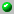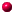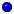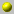Sergey Fomin

The homepage can be accessed here.

Sergey Fomin

Professor of Mathematics, University of Michigan

office: 2858 East Hall
email: fomin@umich.edu
phone: (734) 764-6297
fax: (734) 763-0937

Department of Mathematics
University of Michigan
525 East University Ave.
Ann Arbor, MI 48109-1109, USA

the following papers are available on our server= dvi format= ps format= tex format= pdf formatbalanced_labellings_and_schubert_polynomials
combinatorial_b_n_analogues_of_schubert_polynomialscombinatorial_b_n_analogues_of_schubert_polynomialsdouble_bruhat_cells_and_total_positivityfinite_posets_and_ferrers_shapesgrothendieck_polynomials_and_the_yang_baxter_equationinverting_the_frobenius_mapknuth_equivalence_jeu_de_taquin_and_the_littlewood_richardson_rulemixed_bruhat_operators_and_yang_baxter_equations_for_weyl_groupsnoncommutative_schur_functions_and_their_applicationson_the_number_of_rim_hook_tableauxparametrizations_of_canonical_bases_and_totally_positive_matricesquadratic_algebras_dunkl_elements_and_schubert_calculus
quantum_schubert_polynomialsquantum_schubert_polynomialsrecognizing_schubert_cellsreduced_words_and_plane_partitionsreduced_words_and_plane_partitionsrim_hook_lattices
schubert_polynomials_and_the_nilcoxeter_algebraschur_operators_and_knuth_correspondencessparse_interpolation_of_symmetric_polynomials
total_positivityuniversal_exponential_solution_of_the_yang_baxter_equationyang_baxter_equation_symmetric_functions_and_grothendieck_polynomials

Go back to the directory of pre and reprints.
Or search the database by keywords
Or look for the newest entires in the database